# An Introduction to the Rodin Coil and Vortex-Based Mathematics

An Introduction to the Rodin Coil and Vortex-Based Mathematics

With the introduction of Vortex-Based Mathematics you will be able to see how energy is expressing itself mathematically. This math has no anomalies and shows the dimensional shape and function of the universe as being a toroid or donut-shaped black hole. This is the template for the universe and it is all within our base ten decimal system!

Numbers are Real and Alive not merely symbols for other things. You will discover that the relationships between numbers are not random or man-made but that numbers are actually elementary particles of which everything is composed.

This lost knowledge was well known to our ancients and is now being uncovered for us today. Gradually you will come to see numbers in a simple yet profoundly perfect three-dimensional matrix grid pattern that forms the shape of a torus. The number grid reveals the calibration and timing for an engine that can take us throughout the universe and solve mankind’s energy needs.

As you begin to glide through this explanation of Marko Rodin’s fantastic discoveries, please understand that you must set aside many commonly held belief systems of the way the world works in order to fully comprehend the completely new truths that are embodied in this discovery.

This math truly explains everything that ever was, is, and ever will be in the universe, perfectly, with no omissions. As Russell Blake, senior researcher from Microsoft, has said of Marko’s work, ”
This fantastic coherence has existed since the beginning of time but has yet to be harnessed by mankind and the potential is truly mind-boggling.” Thus, we invite you to charge forward with all your mind, heart and soul into the enchanting realm we like to call The Rodin Experience.

Mathematics as we know it today is only a tool to symbolize quantities. It simply and shamelessly helps the greedy king count his coins.

Vortex-Based Mathematics (VBM) is completely different because it is a dynamic math that shows the relationships and thus the qualities of numbers rather than the quantities.
Within VBM numbers are not symbols that stand for something else, NUMBERS ARE REAL AND ALIVE. But what exactly does that mean you ask, well…

Marko Rodin final conclusion was that all intelligence comes from a person’s name. This led him to understand that not only do our personal names and the language they are spoken in highly affect our personalities but that the most important names are the names of God.

This led Marko into a journey of thoroughly studying all the world’s great religions. When he was introduced to the Baha’i Faith he immediately became a believer.
Literally days later Marko decided to take The Most Great Name of Baha’u’llah
(Prophet of the Baha’i Faith) which is Abha and convert it into numbers.

He did this in an effort to discover the true precise mystical intonation of The Most Great Name of God. Since the Baha’i sacred scripture was originally written in Persian and Arabic Marko used the Abjad numerical notation system for this letter to number translation.

This was a sacred system of allocating a unique numerical value to each letter of the 27 letters of the alphabet so that secret quantum mechanic physics could be encoded into words. What Marko discovered was that (A=1, b=2, h=5, a=1) = 9.

The fact that The Most Great Name of God equaled 9 seemed very important to him as everything he had read in both the Baha’i scriptures and other religious text spoke of nine being the Omni-potent number.

So next he drew out a circle with nine on top and 1 through 8 going around the circle clockwise. Then he discovered a very intriguing number system within this circle.

Marko knew he had stumbled upon something very profound. This circle with its hidden number sequence was the “Symbol of Enlightenment.” This is the MATHEMATICAL FINGERPRINT OF GOD.Follow along as the amazing properties of this symbol unveil themselves to you. Put your pencil on number 1 and without picking up your pencil, move your pencil in a straight line to number 2, then 4, then across the center to 8. Notice that you are doubling. So next should be 16 and it is, but 1+6=7. So move your pencil to 7.

Then 16 doubled is 32, but 3+2=5. So move your pencil to 5. Then 32 doubled is 64 and 6+4=10 and 1+0=1. And you’re back to 1. So move the pencil across the center and back up to 1.

The significance of the Mayan calendar is that 64 is one complete cycle of infinity. Then it begins again with 64 doubled is 128 and 1+2+8=11, then 1+1=2. And so on. You will never get off this track as you keep doubling. Notice the infinity symbol has formed underneath your pencil, creating an ever-repeating pattern of 1, 2, 4, 8, 7, 5. Amazingly, this number sequence stays intact as you half numbers as well. Start again at the 1 but this time go backwards on the infinity symbol.

Half of 1 is .5, so move your pencil to the 5. Then half of .5 is .25, and 2+5=7. So move your pencil to the 7. And half of .25 is .125 and 1+2+5=8. So move to the 8. Next half of .125 is .0625 and 0+6+2+5=13 and 1+3=4. So go across to the 4. And half of .0625 is .03125 and 0+3+1+2+5=11 and 1+1=2. So move to the 2. Forever staying on the route of 1,2,4,8,7,5 even backwards.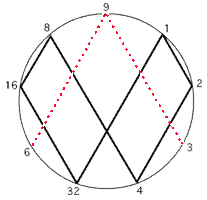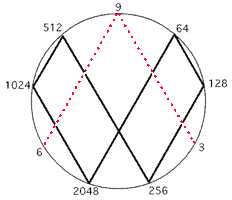DOUBLINGHALVING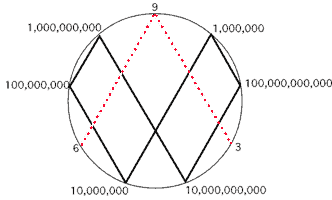POWERS OF TEN

As you replace the decimal numbers with their power (for example 0.5=10, .25=100, 0.125=1,000) you will see that the powers line up horizontally.

The number is on the left, horizontal addition result is on the right. Notice how the 1,2,4,8,7,5 number pattern repeats indefinitely.

 1 = 1 2 = 2 4 = 4 8 = 8 16 = 7 32 = 5 64 = 1 128 = 2 256 = 4 512 = 8 1,024 = 7 2,048 = 5 4,096 = 1 8,192 = 2 16,384 = 4 32,768 = 8 65,536 = 7 131,072 = 5 262,144 = 1 524,288 = 2 1,048,576 = 4 2,097,152 = 8 4,194,304 = 7 8,388,608 = 5 16,777,216 = 1 33,554,432 = 2 67,108,864 = 4 134,217,728 = 8 268,435,456 = 7 536,870,912 = 5 1,073,741,824 = 1 2,147,483,648 = 2 4,294,967,296 = 4 8,589,934,592 = 8

At this point some of you might be thinking, “What in the world do these number patterns have to do with real world applications?” These number groupings piece together into a jig-saw-like puzzle pattern that perfectly demonstrates the way energy flows. Our base-ten decimal system is not man made, rather it is created by this flow of energy. Amazingly, after twenty years of working with this symbol and collaborating with engineers and scientists, Marko discovered that the 1,2,4,8,7,5 was a doubling circuit for a very efficient electrical coil. There was still one more very important number pattern to be realized. On the MATHEMATICAL FINGER PRINT OF GOD notice how the 3, 9, and 6 is in red and does not connect at the base. That is because it is a vector. The 1,2,4,8,7,5 is the third dimension while the oscillation between the 3 and 6 demonstrates the fourth dimension, which is the higher dimensional magnetic field of an electrical coil. The 3, 9, and 6 always occur together with the 9 as the control. In fact, the Yin/Yang is not a duality but rather a trinary. This is because the 3 and 6 represent each side of the Yin/Yang and the 9 is the “S” curve between them. Everything is based on thirds. We think that the universe is based on dualities because we see the effects not the cause.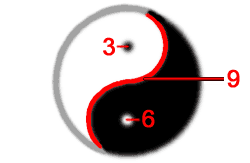When I say the oscillation between the 3 and 6 what I mean is that 3 doubled is 6 and 6 doubled is 12, but 1+2=3, and then 12 doubled is 24, but 2+4=6 and 24 doubled is 48, but 4+8=12 and 1+2=3. So the 3 and 6 go back and forth in their own separate dimension. This creates the other important pattern of (3,9,6,6,9,3,3,9).

Now lay out the number patterns on the 2D grid. Place the 3,9,6,6,9,3,3,9 number pattern in the center of the 1,2,4,8,7,5 number pattern going forward and the 1,2,4,8,7,5 number pattern going backwards this continuation creates an amazingly perfect number map. The MATHEMATICAL FINGER PRINT OF GOD is a legend or blueprint for the torus and can be visualized in a 2D form below.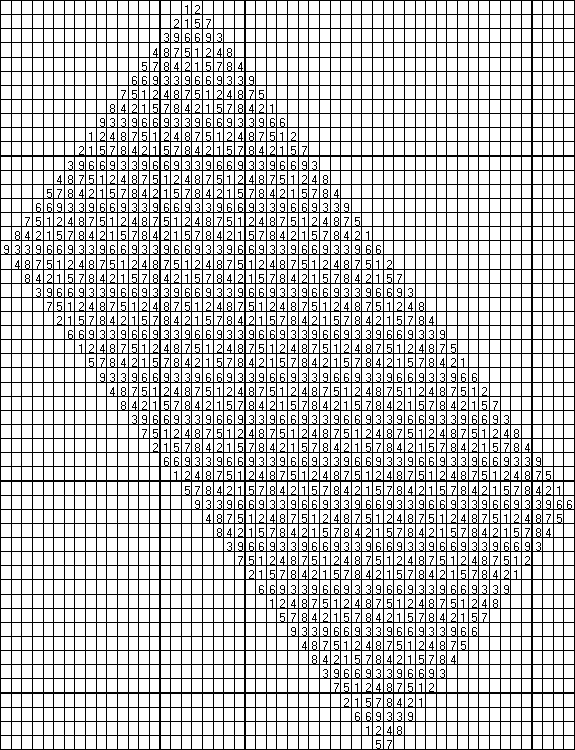The 2D number map forms the 3D skin of the torus and thus demonstrates the concept of the winding of the coil.And the 9 demonstrates the omni dimension which is the higher dimensional flux emanation called Spirit that always occurs within the center of the magnetic field lines. The last number left to be explained from The MATHEMATICAL FINGER PRINT OF GOD is the number 9. The number nine is Energy being manifested in a single moment event of occurrence in our physical world of creation. It is unique because it is the focal center by being the only number identifying with the vertical upright axis. It is the singularity or the Primal Point of Unity. The number nine never changes and is linear. For example all multiples of 9 equal 9. 9×1=9, 9×2=18, but 1+8=9, 9×3=27, but 2+7=9. This is because it is emanating in a straight line from the center of mass out of the nucleus of every atom, and from out of the singularity of a black hole. It is complete, revealing perfection, and has no parity because it always equals itself. The number nine is the missing particle in the universe known as Dark Matter.

The number nine lines up with the center of the infinity symbol and it is from this center that the linear emanations we call Spirit emanate from the center of mass outwards. Spirit is the only thing in the universe that moves in a straight line. Spirit is the inertia aether that Einstein postulated. Spirit is what makes everything else warp and curve around it. The perfect number patterns are actually created by this Spirit energy. Without Spirit the universe would become destitute and void. Spirit flow is the source of all movement as well as the source of the non-decaying spin of the electron.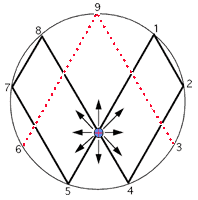Numbers have temporal, spatial and volumetric qualities. For example, physics is the base ten number system. In other words numbers have bilateral or mirror symmetry and line up in stratified layers of horizontal planes. This is the same as physics’ parity. There are nine simple multiplication series (one series for each discrete number 1-9) in Vortex-Based Mathematics. These multiplication series are based upon reducing all products to single digits via horizontal addition forming meaningful number sequences.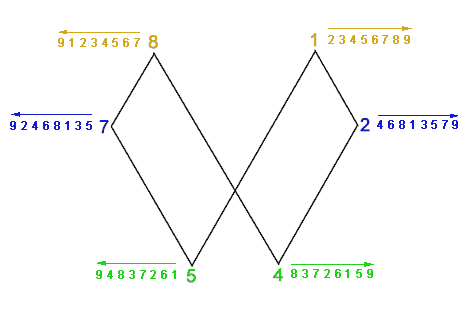There are three polar number pairs; 1 and 8, 2 and 7, 4 and 5. For example, multiples of 4 are 1×4=4, 2×4=8, 3×4=12 but 1+2=3, 4×4=16 but 1+6=7, 5×4=20 but 2+0=2, 6×4=24 but 2+4=6, 7×4=28 but 2+8=10 and 1+0=1, 8×4=32 but 3+2=5, 9×4=36 but 3+6=9. So multiples of 4 equal 4,8,3,7,2,6,1,5 and multiples of 5, the polar opposite of 4, are (5,1,6,2,7,3,8,4). Each pair forms number sequences that are identical but in opposing directions. So if you take the multiples of 5 and look at them running backwards they are the same as the multiples of 4 running forwards. In exactly the same way the multiplication series for the polar pair [1,8] is: (1,2,3,4,5,6,7,8) and (8,7,6,5,4,3,2,1) for the number 1 and the number 8 respectively. The multiplication series for the polar pair [2,7] is: (2,4,6,8,1,3,5,7) and (7,5,3,1,8,6,4,2). Everything is starting from the center outwards, from the singularity.Nature is expressing herself with numbers. The symmetry of our decimal system is a principle of nature. The 9 axis causes the doubling circuit and it is the point towards which matter converges and away from which it diverges or expands. Thus the polar number pairs will be mirror images of each other, both flowing in opposite directions from the central axis. There is perfect symmetry wrapped around a single point coiling outwards the way that petals are wrapped in a rose, or a nautilus shell spirals outward.